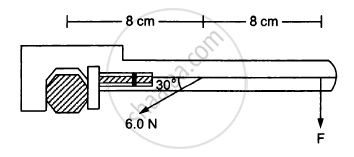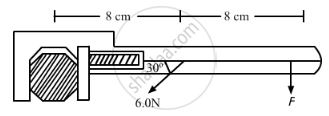Advertisement Remove all ads

# When a Force of 6⋅0 N is Exerted at 30° to a Wrench at a Distance of 8 Cm from the Nut, It is Just Able to Loosen the Nut. - Physics

Sum

When a force of 6⋅0 N is exerted at 30° to a wrench at a distance of 8 cm from the nut it is just able to loosen the nut. What force F would be sufficient to loosen it if it acts perpendicularly to the wrench at 16 cm from the nut?Advertisement Remove all ads

#### SolutionIn the first case,

$\tau_1 = 6\sin30^\circ \times \left( \frac{8}{100} \right)$

In first case,

$\tau_2 = F \times \left( \frac{16}{100} \right)$

To loosen the nut, torque in both the cases should be the same.

Thus, we have

$\tau_1 = \tau_2$

$\Rightarrow F \times \frac{16}{100} = 6\sin30^\circ \times \frac{8}{100}$

$\Rightarrow F = \frac{\left( 8 \times 3 \right)}{16} = 1 . 5 N$

Is there an error in this question or solution?
Advertisement Remove all ads

#### APPEARS IN

HC Verma Class 11, 12 Concepts of Physics 1
Chapter 10 Rotational Mechanics
Q 19 | Page 196
Advertisement Remove all ads

#### Video TutorialsVIEW ALL 

Advertisement Remove all ads
Share
Notifications

View all notifications

Forgot password?